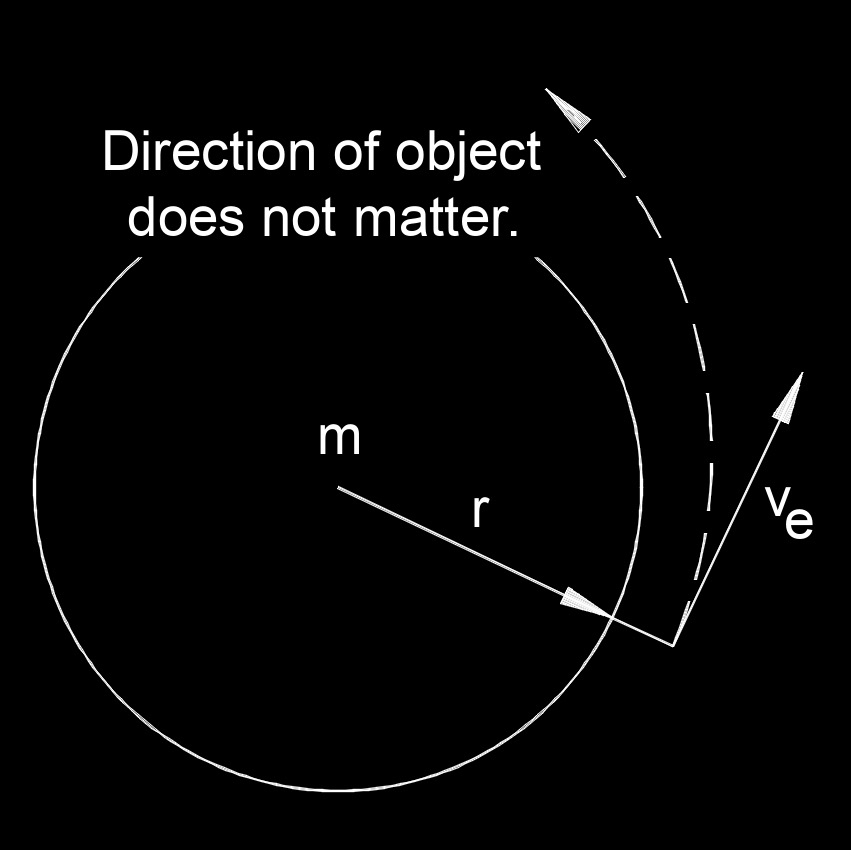# Escape Velocity

Written by Jerry Ratzlaff on . Posted in Classical MechanicsEscape velocity, abbreviated as $$v_e$$, is the minimum velocity required to leave a planet or moon or the minimum velocity to overcome the pull of gravity.

### Escape Velocity formula

$$\large{ v_e = \sqrt { \frac{ 2 \; G \; m}{r} } }$$

$$\large{ v_e = \sqrt { 2 \; g \; r } }$$

Where:

$$\large{ v_e }$$ = escape velocity

$$\large{ g }$$ = gravitational acceleration (9.80665 m/s2 or 32.1740 ft/s2, Earth only)

$$\large{ m }$$ = mass of the plamet or moon

$$\large{ r }$$ = radius from the center of mass (plamet or moon) to start point

$$\large{ G }$$ = universal gravitational constant